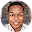# BECE 2000 Integrated Science Paper 2SECTION B ESSAY
1hour [60 marks]

Answer three questions only from this section.
Illustrate your answers wherever possible, with large, clear and fully labelled diagrams. Credit will be given for clarity of expression and orderly presentation of material.
All questions carry equal marks

1. (a) (i) What is personal hygiene?
(ii) List four ways by which personal hygiene could be maintained

2. (a) What is an electrostatic force?

(b) (i) State the unit of
(α) force
(β) work

(ii) A body of mass 80 kg is lifted vertically through a distance of 5.0 m. Calculate the work done on the body.
[Acceleration due to gravity(g)=10 ms-2] (c) Describe briefly how a mixture of common salt and powdered sulphur could be separated. (d) State three characteristics each of
(i) an insect pollinated flower
(ii) a wind pollinated flower

3. (a) (i) What is fertilization

(ii) Describe briefly the processes that lead to fertilization in humans after mating

(b) (i) Define the term density

(ii) Describe briefly how the density of a piece of stone could be determined

(c) (i)What is an element?

(ii) Classify each of the following substances as an element, compound or mixture: Water, air, potassium, alcohol, common salt, sugar

4. (a) Define each of the following terms:
(i) alloy
(ii) diffusion
(iii) colloid

(b) Give two examples each of:
(i) alloy

(ii) colloid

What is pollution?
(ii) List two pollutants each of:
(α) air
(β) water
(γ) land

(d) A coconut of weight 50 N hangs 15 m above the ground.

(i) Name the type of energy possessed by the coconut.

(ii) Calculate the value of this energy